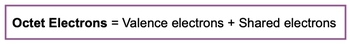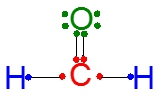## General Chemistry

Learn the toughest concepts covered in Chemistry with step-by-step video tutorials and practice problems by world-class tutors

11. Bonding & Molecular Structure

# Octet Rule

The Octet Rule states that main-group elements will generally form enough bonds to obtain 8 electrons in their valence shell.

The Octet Rule
1
concept

## Octet Rule1m
Play a video:
when you hear the term optic role, just realize it's the tendency of most man group elements and achieving eight octet electrons by way of chemical bonding. Now we're going to say they do so toe toe, have the same number of octet electrons as a noble gas and realize that each co violent bond formed between two elements equals the sharing up to valence electrons between them. Now we talked about octet electrons. Now valence. Electrons are electrons and element possesses based on group number. So aluminum being in Group 38 means it has three valence electrons now shared electrons are the electrons and element gains through a chemical bond. And when we talk about opted electrons, opted electrons equals these valence electrons, plus your shared electrons in. By combining the violence and shared, this justifies the need to get to eight total octane electrons for a majority of the main group elements. Remember, hydrogen doesn't fit this definition neatly. It only needs one valence electron to get the noble gas configuration off helium. So we don't talk about hydrogen when it comes to the octet rule. Now that we've learned about these three different classes of electrons, click on to the next video and let's take a look at an example question2
example

## Octet Rule Example 11m
Play a video:
in this example question, it says which the following statements is true in terms of the following compound. In the following compound, we have a nitrogen atom in the center and it is surrounded by three florins. Realize here that nitrogen it's a maghrib element, and the drive of man group elements is trying to get to eight octet electrons. So that means that the answer could be B or C. Now we could look at its valence electrons. Its valence electrons are the electrons tied to its group number. We're gonna say nitrogen and Group five A. So it should have five valence electrons. These air the electrons that brings to the table in order to contribute to this chemical compound, so nitrogen and group five days. So this is 12345 electrons it's brought in. The shared electrons are the electrons that it gains from chemical bonding to flooring atoms. So we'd say here that it's shared electrons. If we take a look, it is gaining additional electrons from the flooring atoms, and that five plus three is what gives us eight octet electrons. So here the best answer would be option B. So just keep in mind, elements come in with their electrons and to in order to get to the eight opted electrons, they can share electrons from the surrounding elements around them.
3
Problem

How many shared electrons are around the oxygen atom?a) 4

b) 2

c) 6

d) 10

e) 8

4
Problem

Which of the following atoms has the most valence electrons?

a) O

b) Be

c) He

d) N

e) Si

5
concept

## Octet Rule2m
Play a video:
now, how could it be chemistry? Without some exceptions, we learned that a majority of the main group elements wanna have eight octet electrons around themselves to resemble more like the noble gasses. But now we're going to see that some elements can have a non octet number of electrons and be completely fine and stable. So we're gonna say these elements are stable with a non octet number of electrons. We talk about incomplete octet and expanded octet. It's in an incomplete octet. An element is able to maintain its stability when having less than eight octet electrons around itself and in an expanded octet. Another element is able to maintain the stability when it has mawr than eight octet around octet electrons around itself. Now we're going to say here that they're non octet. Number of electrons typically is twice there. Group number. So if we take a look, if we're looking at a group to element twice, so two times two, we mean that it's okay with having four electrons for its number of octane electrons. Here, Group three is okay with having six octet electrons, 10 octet electrons for six On Friday, 12 octet electrons from element in Group six A. And then we have 14 and then 16. We take a look here. The elements that typically have a nen complete op tech are hydrogen, helium, beryllium and then in Group three, A's boron, aluminum, gallium and indium, the elements that typically can have an expanded octet. They are found in row three or period three and lower. So we're talking about phosphorous, sulfur, chlorine, arsenic, selenium, bro, mean crypt on delirium, iodine, xenon and radon. I remember noble gasses are very stable. They tend not to want to get involved in bonding preferences because they're perfect, but these ones that are lowered down since there is so much lower they have larger shells so they can form bonds. Um, if needed, and just realize here that when it comes to their non octet number, they're not gonna have eight octet electrons. They tend to have this twice the value of their group number. So keep that in mind when examining these different types of elements that have non octet number off electrons

Some elements can have less or more than 8 octet electrons around them while still maintaining stability.

6
example

## Octet Rule Example 21m
Play a video:
here. It says how maney octet electrons around the phosphorus atom in the following compound. So remember your octet electrons equals your valence electrons, plus your shared electrons. If we take a look here, your valence electron number is based on your group number. Phosphorus is in group five A. So it has five valence electrons. So 12345 and then the shared electrons are the electrons you gain by forming a chemical bond with another element. So here are shared. Electrons would be the ones that were gaining from forming a bond with the hydrogen atoms. So 12 34 and five. So here we see that phosphorus has 10 total octet electrons here. This makes sense because we said that when we're dealing with a non octet number of electrons, it's typically twice the group number of the element Phosphorus is in Group five A, and if you multiply that by two, we typically concealed go all the way up to 10 valence electrons. So that means option B would be are correct choice
7
Problem

Which of the following contains an atom that may have an incomplete octet?

a) CCl4

b) NCl3

c) OCl2

d) SeCl6

e) BCl3